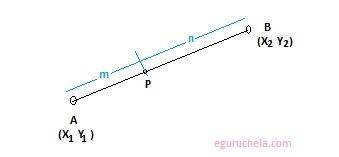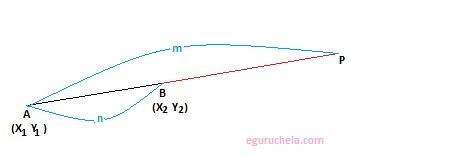### Coordinates of Points Externally/Internally Calculator

Calculator find the coordinates of point p which divides the line joining two Entered points A and B internally or externally, in a specified ratio m and n. Coordinates of point is a set of values that is used to determine the position of a point in a two dimensional plane.
 X1: Y1: X2: Y2: Ratio m: n:
 Externally Internally

 Coordinates of Point

#### Internally divided line segment:

The given coordinates forms a line AB where point P(xp, yp) lies outside of the line segment AB. It can divide the AB externally as showing below:The formula used for internally division of a line segment as follows: $$( \frac {mx_{\,2} + nx_{\,1} }{m + n},\frac {my_{\,2} + ny_{\,1} }{m + n} )$$

#### Externally divided line segment:

The given coordinates forms a line AB where point P(xp, yp) lies on the line segment AB joining Point P. It is showing bellow:The formula used for externally division of a line segment as follows: $$( \frac {mx_{\,2} - nx_{\,1} }{m - n},\frac {my_{\,2} - ny_{\,1} }{m - n} )$$